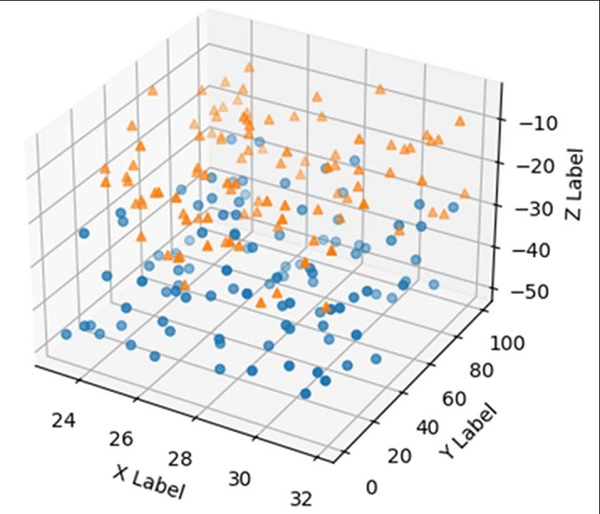# How to make a 3D scatter plot in Python?

To get a 3D plot, we can use fig.add_subplot(111, projection='3d') method to instantiate the axis. After that, we can use the scatter method to draw different data points on the x, y, and z axes.

## Steps

• Create a new figure, or activate an existing figure.

• Add an ~.axes.Axes to the figure as part of a subplot arrangement, where nrows = 1, ncols = 1, index = 1 and projection is ‘3d’.

• Iterate a list of marks, xs, ys and zs, to make scatter points.

• Set x, y, and z labels using set_xlabel, y_label, and z_label methods.

• Use plt.show() method to plot the figure.

## Example

import matplotlib.pyplot as plt
import numpy as np

np.random.seed(1000)

fig = plt.figure()

n = 100

for m, zl, zh in [('o', -50, -25), ('^', -30, -5)]:
xs = (32 - 23) * np.random.rand(n) + 23
ys = (100 - 0) * np.random.rand(n)
zs = (zh - zl) * np.random.rand(n) + zl
ax.scatter(xs, ys, zs, marker=m)

ax.set_xlabel('X Label')
ax.set_ylabel('Y Label')
ax.set_zlabel('Z Label')

plt.show()

## Output